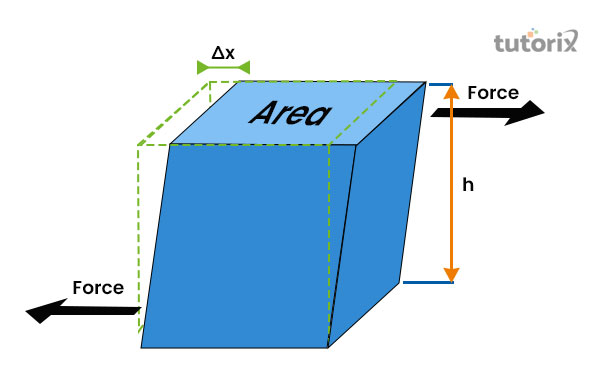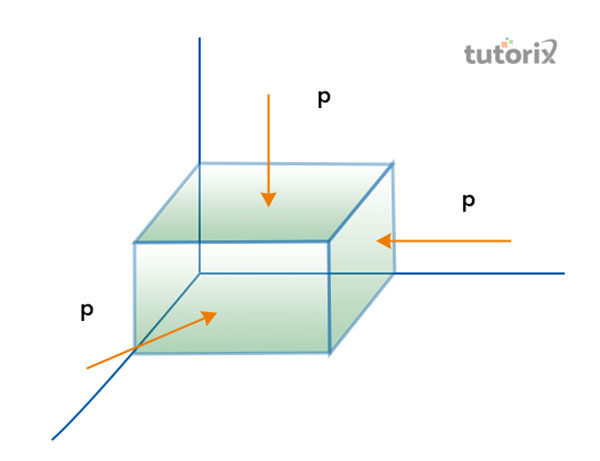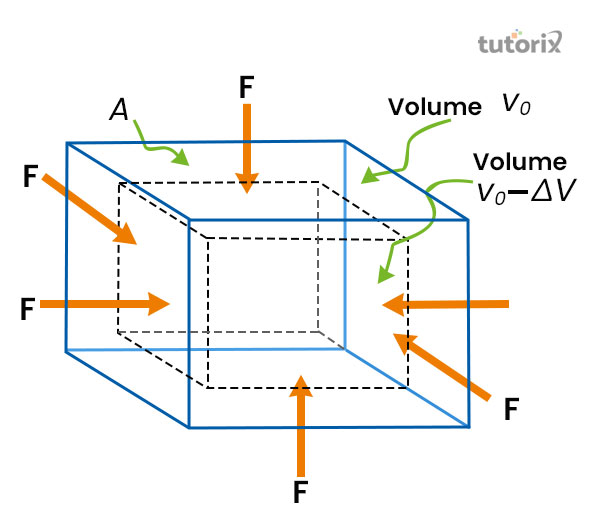# Relation between Elastic Constants

## Introduction

According to physics, emanating a connection among all the three elastic constants can result in the appropriate interrelation among all of them known as the formula of the “Elastic constant”. Based on the statement of the “young’s modulus” (E) and the “Poisson ratio (𝝂)” are understood as the liberated “elastic constants” they can be transmitted by performing the investigations properly. In this tutorial, the relation between the “elastic constants” is mentioned.Figure 1: “Elastic Constant”

## Elastic Limit

In a situation where an external force can make act on a body, it gets tended to experience deformation. This theory in physics is known as the “elastic body”. Additionally, these belongings, by the integrity of which specific item returns to its foremost position after the displacement of the external power, is named elasticity. Based on this situation, the body can reconstruct the older size and shape when the deformation is caused due to the application of an external force within a limit (Lkouniv.ac, 2022). Additionally, the limit of vale can force the deformation to make the complete disappear depending upon the displacement of the applied power. Further, the force’s value is approximating controlling the limitation of force which is identified as the limit of the item’s elasticity.

## Elastic Constant Formula

The proportional amount of strain gets produced when an elastic body is creating stress. The ratio of stress that is applied to generate strains will continue to develop constant and it is comprehended in physics as the “elastic constant”. The behaviour of elasticity of an item is understandable by the “elastic constant” (Theconstructor, 2022). The most useable formula of the “elastic constant” is depending on the three different modules. The basic formula, which is used to comprehend the “elastic constant”, is

$$\mathrm{E =\frac{Stress}{Strain}}$$

## Different Elastic Constants

The connection and the formula of the “elastic constants” is depending applied stress to produce strain. The module of elasticity is classified into the following three types:

### “Young’s Modulus (E)”

According to the law constructed by Hooke, when a body is about to tensile pressure or stress, the applied stress is instantly proportional to the pressure within the “elastic limits” of that body. The applied ratio of stress is regarded with the strain and it is persistent. This application and formula are known as the modulus of Young’s or the “elasticity modulus” (Hasan, Rosli & Alkahari, 2020). The “Young’s modulus” is comprehended by the “E” letter.

### “Bulk Modulus (K)”

The corresponding value of stress can be found as constant when the body is mutually vertical and manage to produce direct stress. According to the law, the definition of the constant elasticity is depending on the direct stress to the connected volumetric strain encountered to be consistent. The “Bulk Modulus” ratio is connected by the representation of the letter “K’. Calculating the “elasticity modulus” with the help of K is possible by the application of the formula:

$$\mathrm{K = \frac{direct\: stress}{volumetric \:strain}}$$

(Yuan et al. 2019). The critical note of the modulus is it can be expressed with the understanding knowledge of an item which can resist the deformation in modification in the volume at the time when it can make changes under the stresses.

### Rigidity Modulus

The modulus of the rigidity is also comprehended as the “Shear modulus” and it is useful to define the measure of the stiffness of an item’s elasticity. According to this module, the body shape altered when the corresponding shear stress is applied to the body. The “G” letter represents the “Unit of rigidity”.Figure 2: Engineering References to “Elastic Constant”

## Relationship between Elastic Constants

“Elastic constants” is one the vital most topic in physics which can be explained by the application of the three modules, which are the “Young’s, Bulk, and Rigidity”.

Understanding the stress and strain is vital to comprehending the connection to the formation of the “elastic constants” of an item. Deforming force is performing on a solid that will consequence the transformation in its actual dimension. In such circumstances, the relation between elastic constants can be applied to comprehend the deformation’s magnitude (Mechcontent, 2022). When the force coming from an external application is removed, the body can go back to the source and make the original size and shape.Figure 3: Elasticity: Strain and Stress

## Hooke’s law

The law of Hooke describes that when an item is packed within the boundary of elasticity where the stress is symmetrical to the strain, it is able to construct by the stress. This also refers to the stress ratio to the connection with the value of the strain which is persistent within the boundary of elasticity.

Hence, the formula of a constant is $\mathrm{\frac{Stress}{Strain}= Constant}$

## Conclusion

In this tutorial, a comprehensive view has been developed upon the deformation force which is useful in the application of gaining knowledge about the stress and strain. Moreover, the developed factors of the tutorial are focusing on the different modulus that is connected to the understandable knowledge of the “elastic constants”. As the elasticity of an item is depending on the applied stress and strain, it can be varied to the different ratios that are applied in the relation to the elasticity and limit of an item.

Q1. What are the “elastic constants”?

Ans. The “elastic constants” are the constant that helps explain the connection between the involved classes of stress and the strain that is also corresponding to it.

Q2. What is the “elastic constant K”?

Ans. The rate of “spring constant” is represented with the letter K in physics. This is also referred to as the displaying variation of the behaviour that is reliable to the linear line.

Q3. What is known as the “modulus of Bulk” in elasticity?

Ans. The “Bulk modulus” is referred to as the process of measuring the amount of reduced volume with the increment of pressure. This function is widely relying on the temperature.

## References

### Journals

Hasan, R., Rosli, N. A., & Alkahari, M. R. (2020). Elastic modulus approximation via experimental formulation for ABS lattice structure material. Proceedings of Mechanical Engineering Research Day, 2020, 51-52. Retrieved from: https://www3.utem.edu.my

Yuan, X., Zhu, X., Wang, C., & Zhang, L. (2019). Research on theoretical model of dynamic bulk modulus of gas-containing hydraulic oil. IEEE Access, 7, 178413-178422. Retrieved from: https://ieeexplore.ieee.org/iel7/6287639/8600701/08931608.pdf

### Websites